Next: 4.3.2 Assembly of the Up: 4.3 Simulation in FEDOS Previous: 4.3 Simulation in FEDOS

## 4.3.1 Newton's Method

The non-linear system of equations, which results from the discretization described in Section 4.2, is solved in FEDOS using the conventional Newton method [153,154]. Generally, a non-linear system of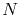equations is expressed as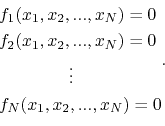If a given solution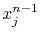,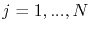, is known, these equations can expanded in the vicinity ofusing Taylor's series, which to the first order are approximated by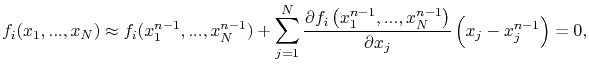(4.69)

for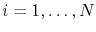. The system of equations (4.69) is written in matrix form as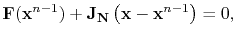(4.70)

where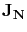is the so-called Jacobian matrix, for which the entries are given by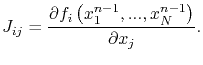(4.71)

Thus, the solution of (4.70) is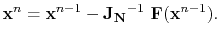(4.72)

In FEDOS, instead of using (4.72), the linear system of equations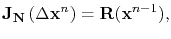(4.73)

is assembled and solved, so that the increments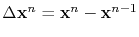are determined. Here,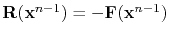is called residual. In this way, the new approximate solution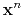is updated according to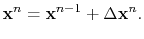(4.74)

These calculations are performed as an iterative process, where the solution of the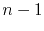iteration is used to compute the new solution. The accuracy of the new approximate solution is controlled by the conditions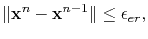(4.75)

where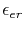is a given tolerance for the solution error, and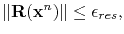(4.76)

whereis a given tolerance for the residual and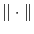represents the Euclidean norm. The iterative procedure is terminated, if both criteria are fulfilled.Next: 4.3.2 Assembly of the Up: 4.3 Simulation in FEDOS Previous: 4.3 Simulation in FEDOS

R. L. de Orio: Electromigration Modeling and Simulation